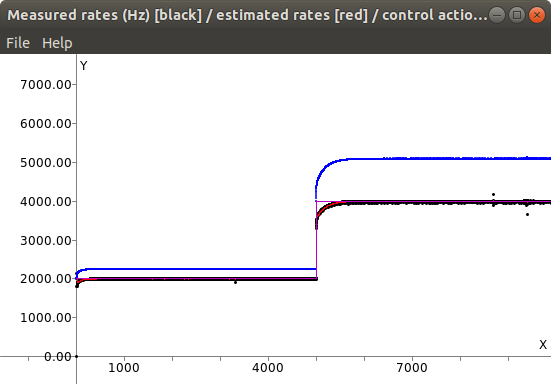# class mrpt::system::CControlledRateTimer

A class for calling sleep() in a loop, such that the amount of sleep time will be computed to make the loop run at the desired rate (in Hz).

This class implements a PI controller on top of a vanilla CRateTimer object, ensuring a high accuracy in achieved execution rates. Note that this is done by setting a slightly-higher rate (“control action”) to the internal CRateTimer, such that the error between the user-provided expected rate and the actual measured rate (low-pass filtered) is decreased by means of a PI controller.

Note that rates higher than a few kHz are not attainable in all CPUs and/or kernel versions. Find below some graphs illustrating how this class tries to achieve a constant setpoint rate (given by the user), reacting to changes in the setpoint values:This graphs is generated with the example:

`system_control_rate_timer_example --rate1 2000.0 --rate2 4000.0`

All the parameters for the PI controller and low-pass filter (rate estimator) are settable by the user to adapt them to specific needs.

Control law by Francisco Jose Mañas Alvarez

[New in MRPT 2.0.4]

```#include <mrpt/system/CControlledRateTimer.h>

class CControlledRateTimer: public mrpt::system::COutputLogger
{
public:
// construction

CControlledRateTimer(const double rate_hz = 1.0);

//
methods

void setRate(const double rate_hz);
bool sleep();
double controllerParam_Kp() const;
void controllerParam_Kp(double v);
double controllerParam_Ti() const;
void controllerParam_Ti(double v);
double lowPassParam_a0() const;
void lowPassParam_a0(double v);
double followErrorRatioToRaiseWarning() const;
void followErrorRatioToRaiseWarning(double v);
double actualControlledRate() const;
double estimatedRate() const;
double estimatedRateRaw() const;
};```

## Inherited Members

```public:
// structs

struct TMsg;```

## Construction

`CControlledRateTimer(const double rate_hz = 1.0)`

Ctor: specifies the desired rate (Hz)

## Methods

`void setRate(const double rate_hz)`

Changes the object loop rate (Hz)

`bool sleep()`

Sleeps for some time, such as the return of this method is 1/rate (seconds) after the return of the previous call.

Returns:

false if the rate could not be achieved (“we are already late”), true if all went right.

`double controllerParam_Kp() const`

PI controller Kp parameter [default=1.0].

`double controllerParam_Ti() const`

PI controller Ti parameter [default=0.0194].

`double lowPassParam_a0() const`

Low-pass filter a0 value [default=0.9]: estimation = a0*input + (1-a0)*former_estimation.

`double followErrorRatioToRaiseWarning() const`

Get/set ratio threshold for issuing a warning (via COutputLogger interface) if the achieved rate is not this close to the set-point [Default=0.2, =20%].

`double actualControlledRate() const`

Gets the actual controller output: the rate (Hz) of the internal CRateTimer object.

`double estimatedRate() const`

Gets the latest estimated run rate (Hz), which comes from actual period measurement, low-pass filtered.

`double estimatedRateRaw() const`

Last actual execution rate measured (Hz), without low-pass filtering.